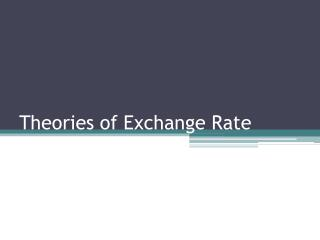# Theories of Exchange Rate - PowerPoint PPT PresentationDownload PresentationTheories of Exchange Rate

Theories of Exchange Rate
Download Presentation## Theories of Exchange Rate

- - - - - - - - - - - - - - - - - - - - - - - - - - - E N D - - - - - - - - - - - - - - - - - - - - - - - - - - -
##### Presentation Transcript

1. Theories of Exchange Rate

2. Introduction • An exchange rate is the relative price of one currency in terms of another. • It influences allocation of resources within and across countries.

3. Introduction • Exchange rates are affected by many factors. • For instance, Balance of payments, inflation, interest rates, money supply, political factors, market sentiments, technical factors etc. • Important ones are Price and Interest rates

4. Theories of Exchange Rate The three theories of exchange rate determination are- • Purchasing Power Parity (PPP), which links spot exchange rates to nations’ price levels. • The Interest Rate Parity (IRP), which links spot exchange rates, forward exchange rates and nominal interest rates. • The International Fisher Effect (IFE) which links exchange rates to nations’ nominal interest rate levels.

5. Purchasing Power Parity(PPP) • ThePPPtheoryfocusesontheinflation-exchangeraterelationships.Ifthelawofonepriceweretrueforallgoodsandservices,wecouldobtainthetheoryof PPP.Therearetwo formsofthePPPtheory: • Absolute form of PPP & • Relative form of PPP

6. Absolute Purchasing Power Parity(PPP) • TheabsolutePPPtheorypostulatesthattheequilibriumexchangeratebetweencurrenciesoftwocountriesIsequaltotheratioofthepricelevelsinthetwonations.Thus,pricesofsimilarproductsoftwodifferentcountriesshouldbeequalwhenmeasuredinacommoncurrencyaspertheabsoluteversionofPPPtheory. (The Law of One price)

7. RelativePurchasingPower Parity • Therelativeform ofPPPtheoryisanalternativeversionwhichpostulatesthatthechangeintheexchangerateoveraperiodoftimeshouldbeproportionaltotherelativechangeInthepricelevelsinthetwonationsoverthesametimeperiod.

8. Interest Rate Parity (IRP) • A forward exchange rate is the rate that is currently paid for the delivery of a currency at some future date. • It has the spot rate as its base, plus the interest factor. • The interest factor which is factored into the rate is called the interest rate parity.

9. InternationalFisherEffect(IFE) • The FE theory suggests that foreign currencies with relatively high interest rates will depreciate because the high nominal interest rates reflect expected inflation.

10. Which factors affect Exchange rates? Which factor is the main? • What is the difference between absolute and relative PPP? • What is the meaning of The Low of One Price?

11. Thank you for attention!